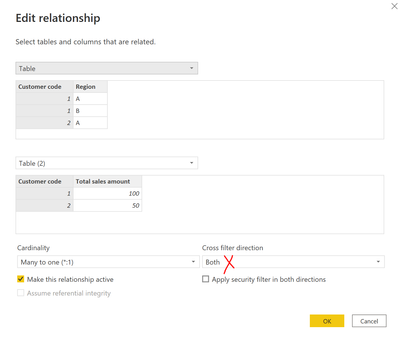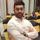cancel
Showing results for
Did you mean:Frequent Visitor

## SUM doesn't filter on region

I have 2 tables:

Transactions:

 Customer code Region Other columns .... 1 A 1 B 2 A

Total sales per customer:

 Customer code Total sales amount 1 100 2 50

I want to create a diagram that show the total per region:

A = 150

B = 100

What I get is:

A = 250

B = 250

The tables are connected on Customer code using many (Transactions) to 1 (Total sales per customer).

The diagram view uses:
- Row: Region column

- Value: SUM([Total sales amount])

How can I solve this?

1 ACCEPTED SOLUTIONSuper User

The reason that you're getting the same result for every row is that the filter cannot pass from the Transactions table to the Total Sales Per Customer because it is a many-to-one relationship. You will need to iterate over the transactions table and sum the related value from the Total Sales Per Customer table

`Total Sales =SUMX( Transactions, RELATED('Total Sales Per Customer'[Total Sales amount]) )`
4 REPLIES 4Super User

Change the Cross filter direction to the Both and that will sort out your issue.If this post helps, please consider accepting it as the solution to help the other members find it more quickly.Super User

The reason that you're getting the same result for every row is that the filter cannot pass from the Transactions table to the Total Sales Per Customer because it is a many-to-one relationship. You will need to iterate over the transactions table and sum the related value from the Total Sales Per Customer table

`Total Sales =SUMX( Transactions, RELATED('Total Sales Per Customer'[Total Sales amount]) )`Frequent Visitor

SUMX is the iterative function which performs on row-level.

Sales = SUMX([Total sales amount])Frequent Visitor

Following are the two methods using CROSSFILTER and RELATED dax functions.